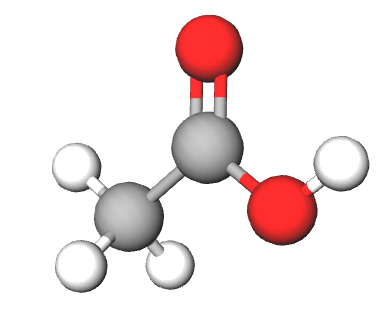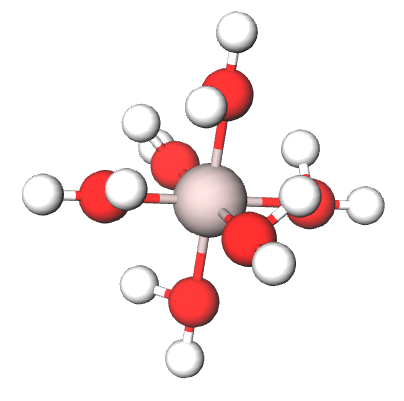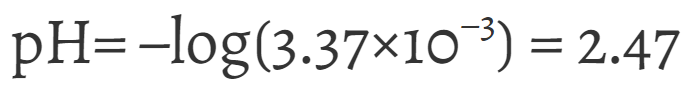# 14.3: The pH of Solutions of Weak Acids

When any weak acid, which we will denote by the general formula HA, is dissolved in water, the reaction

$\text{HA} + \text{H}_{2}\text{O}\rightleftharpoons \text{H}_{3}\text{O}^{+} + \text{ A}^{-}$

proceeds to only a limited extent, and we must allow for this in calculating the hydronium-ion concentration and hence the pH of such a solution. In general the pH of a solution of a weak acid depends on only two factors, the concentration of the acid, ca, and the magnitude of an equilibrium constant Ka, called the acid constant, which measures the strength of the acid. The acid constant is defined by the relationship:

$K_{c}\text{ }\times \text{ 55.5 mol L}^{-1}=K_{a}=\frac{[\text{ H}_{3}\text{O}^{+}][\text{ A}^{-}]}{[\text{ HA }] }$

(The acid constant is Kc multiplied by the constant concentration of water, as already defined in the section on the law of chemical equilibrium. It is also called the ionization constant or the dissociation constant of the acid.)

In Example 5 on The Law of Chemical Equilibrium we showed how measurements of the conductivity of acetic acid solutions could be used to find the acid constant for acetic acid, Ka(CH3COOH). You will recall from that example that Ka was only approximately a constant, varying from a value of 1.81 × 10–5 mol/L in very dilute solutions to a value of 1.41 × 10–7 mol/L in a 1M solution. A similar variation is found for other weak acids, so that most of the calculations we do using Ka are only approximate. Only two or possibly three significant figures should be retained.

The table below gives the Ka values for a few selected acids arranged in order of their strength. This table is part of our larger collection of acid and base resources. It is at once apparent from this table that the larger the Ka value, the stronger the acid. The strongest acids, like HCl and H2SO4 have Ka values which are too large to measure, while another strong acid, HNO3, has Ka value close to 20 mol/L. Typical weak acids such as HF and CH3COOH have acid constants with a value of 10–4 or 10–5 mol/L. Acids like the ammonium ion, NH4+, and hydrogen cyanide, HCN, for which Ka is less than 10–9 mol/L are very weakly acidic.

You may notice that some acids have multiple Ka values. This is due to the fact they are polyprotic, which means they are able to give up more than one proton. However, due to molecular forces, the ease with which each proton gives up its protons varies, giving different K values for each proton given up.

Table $$\PageIndex{2}$$ The Acid Constants for Some Acids at 25°C.
Acid Formula and Ionization Equation Ka Molecular Shape
$$\text{Acetic}$$

$$\ce{CH3COOH + H2O <=> H3O^+ +CH3COO^-}$$

$$1.8 \times 10^{-5}$$$$\text{Aluminum ion}$$ $$\ce{ Al(H2O)6^{3+} + H2O <=> H3O^+ + Al(H2O)5OH^{2+} }$$ $$7.2 \times 10^{-6}$$$$\text{Ammonium ion}$$ $$\ce{NH4^+ + H2O <=> H3O^+ + NH3}$$ $$5.6 \times 10^{-10}$$$$\text{Hydrochloric}$$ $$\ce{HCl + H2O -> H3O+ + Cl^-}$$ $$\text{very large}$$$$\text{Hydrogen peroxide}$$ $$\ce{H2O2 + H2O <=> H3O^+ + HO2^-}$$ $$2.1 \times10^{-12}$$$$\text{Phosphoric}$$

$$\ce{H3PO4 + H2O <=> H3O^+ + H2PO4^-}$$

$$\ce{H2PO4^- + H2O <=> H3O^+ + HPO4^{2-}}$$

$$\ce{HPO4^{2-} + H2O <=> H3O^+ + PO4^{3-}}$$

$$K_1 = 7.2 \times10^{-3}$$

$$K_2 = 6.3 \times10^{-8}$$

$$K_3 = 4.6 \times10^{-13}$$$$\text{Sulfuric}$$

$$\ce{H2SO4 + H20 <=> H3O^+ + HSO4^-}$$

$$\ce{HSO4^- + H20 <=> H3O^+ +SO4^{2-}}$$

$$K_1 = \text{very large}$$

$$K_2 = 1.1\times 10^{-2}$$• Taken from Hogfelt, E. Perrin, D. D. Stability Constants of Metal Ion Complexes, 1st ed. Oxford; New Pergamon, 1979-1982. International Union of Pure and Applied Chemistry, Commission on Equilibrium. ISBN: 008020958

Before we can go on to discuss how the hydronium-ion concentration and the pH of a solution of a weak acid depend on the concentration of the acid, we need to clarify a point of terminology. In order to do this let us take as an example a 0.0010 M solution of acetic acid. Conductivity measurements discussed in the section on weak acids in reactions in aqueous solutions show that only about 10 percent of the acid molecules have donated protons to water at any given time. We thus have a situation which can be summarized schematically in the following way:

$\underset{\text{0.0009 mol L}^{-1}}{\overset{\text{90 }\%\text{ }}{\mathop{\text{CH}_{3}\text{COOH}}}}\,\text{ + H}_{2}\text{O } \rightleftharpoons \text{ }\underset{\text{0.0001 mol L}^{-1}}{\overset{\text{10 }\%\text{ }}{\mathop{\text{CH}_{3}\text{COO}^{-}}}}\,\text{ + }\underset{\text{0.0001 mol L}^{-1}}{\mathop{\text{H}_{3}\text{O}^{\text{+}}}} ~~~~~~~$

In such a solution there is some ambiguity as to what we mean by the phrase concentration of acetic acid. Do we mean 0.0010 mol L–1, or do we mean 90 percent of this value, namely, 0.0009 mol L–1? In order to resolve this difficulty, we will use the term stoichiometric concentration of acid and the symbol ca to indicate the quantity 0.0010 mol L–1, that is, to indicate the total amount of acetic acid originally added per unit volume of solution. On the other hand we will use the term equilibrium concentration

and the symbol [CH3COOH] indicate the quantity 0.0009 mol L–1 that is, the final concentration of this species in the equilibrium mixture.

Let us now consider the general problem of finding [H3O+]in a solution of a weak acid HA whose acid constant is Ka and whose stoichiometric concentration is ca. According to the equation for the equilibrium,

$\text{HA} + \text{H}_{2}\text{O}\rightleftharpoons \text{H}_{3}\text{O}^{+} + \text{ A}^{-}$

for every mole of H3O+ produced, there must also be a mole of A produced. At the same time a mole of HA and a mole of H2O must be consumed. Since the volume which all these species occupy is the same, any increase in [H3O+] must be accompanied by an equal increase in [A] and an equal decrease in [HA]. Consequently we can draw up the following table (in which equilibrium concentrations of all species have been expressed in terms of [H3O+]:

Species HA H3O+ A
Initial concentration (mol/L) ca 10–7 0

Change in concentrationa

(mol/L)

–[H3O+] [H3O+] [H3O+]

Equilibrium concentration

(mol/L)

ca –[H3O+] [H3O+] [H3O+]

aThe hydronium-ion concentration actually increases from 107 mol/L to the equilibrium concentration, and so the change in each of the concentrations is ± ([H3O+ ] –10-7 mol/L). However, the concentration of hydronium ions produced by the weak acid is usually so much larger than –10-7 mol L-1 that the latter quantity can be ignored. In the case of 0.0010 M acetic acid, for example, [H3O+ ] ≈ 1 × 10-4 mol/L. Subtracting gives:

\begin{align} & \text{ 0.000 100 0 mol L}^{-1} \\ - & \underline{​\text{0.000 000 1 mol L}^{-1}} \\ & \text{0.000 099 9 mol L}^{-1} \end{align}

which is very close to 1 × 10-4 mol/L.

We can now substitute the equilibrium concentrations into the expression

$K_{a}=\frac{\text{ }\!\![\!\!\text{ H}_{\text{3}}\text{O}^{\text{+}}\text{ }\!\!]\!\!\text{ }\!\![\!\!\text{ A}^{-}\text{ }\!\!]\!\!\text{ }}{\text{ }\!\![\!\!\text{ HA }\!\!]\!\!\text{ }}=\frac{\text{ }\!\![\!\!\text{ H}_{\text{3}}\text{O}^{\text{+}}\text{ }\!\!]\!\!\text{ }^{\text{2}}}{c_{a}-\text{ }\!\![\!\!\text{ H}_{\text{3}}\text{O}^{\text{+}}\text{ }\!\!]\!\!\text{ }}\text{ }\label{8}$

This could be solved for [H3O+] by means of the quadratic formula, but in most cases a quicker approximate method is available. Since the acid is weak, only a small fraction of the HA molecules will have donated protons to form H3O+ ions. Therefore [H3O+] is only a small fraction of ca and can be ignored when we calculate ca – [H3O+]. That is,

$c_{a} ​– \text{[H}_{3} \text{O}^{+} ] \approx c_{a} ~~~~~~~~~ \text{(5)}$

Equation $$\ref{8}$$ then becomes

$K_{a}= \frac{\text{ [} \text{ H}_{\text{3}}\text{O}^{\text{+}}\text{ }]\text{ }^{\text{2}}}{c_{a}}$

which rearranges to

$\text{[H}_{3} \text{O}^{+}]^{2} \approx K_{a}c_{a}$

Taking the square root of both sides gives an important approximate formula:

$\text{[H}_{3} \text{O}^{+} ] \approx \sqrt{K_{a}c_{a}} ~~~~~~~\label{12}$

Example $$\PageIndex{1}$$: pH Calculation

Use Equation $$\ref{12}$$ to calculate the pH of a 0.0200-M solution of acetic acid. Compare this with the pH obtained using the [H3O+] of 5.92 × 10–4 mol L–1 derived from accurate conductivity measurements.

Solution

From first table above, Ka = 1.8 × 10–5 mol L–1. Since ca = 2.00 × 10–2 mol L–1, we have

\begin{align} \text{ }\!\![\!\!\text{ H}_{\text{3}}\text{O}^{\text{+}}\text{ }\!\!]\!\!\text{ }=\sqrt{K_{a}c_{a}} \\ \text{ }=\sqrt{\text{(1}\text{.8 }\times \text{ 10}^{-\text{5}}\text{ mol L}^{-\text{1}}\text{)(2}\text{.00 }\times \text{ 10}^{-\text{2}}\text{ mol L}^{-\text{1}}\text{)}} \\\text{ }=\sqrt{\text{3}\text{.6 }\times \text{ 10}^{-\text{7}}\text{ mol}^{\text{2}}\text{ L}^{-2}}=\sqrt{\text{36 }\times \text{ 10}^{-8}\text{ mol L}^{-\text{1}}} \\\text{ }\!\![\!\!\text{ H}_{\text{3}}\text{O}^{\text{+}}\text{ }\!\!]\!\!\text{ }=\text{6}\text{.0 }\times \text{ 10}^{-\text{4}}\text{ mol L}^{-\text{1}} \\\text{ pH}=-\text{log(6}\text{.0 }\times \text{ 10}^{-\text{4}}\text{)}=-\text{(0}\text{.78}-\text{4)}=\text{3}\text{.22} \\\end{align}

Using the accurate [H3O+] from conductivity measurements,

$$\text{pH} = – \text{log} (5.92 \times 10^{-4}) = –(0.772 – 4) = 3.228$$

Note that the approximate equation gives an [H3O+] which differs by 1 in the second significant digit from the accurate value. The calculated pH differs by 1 in the second place to the right of the decimal―roughly the same as the accuracy of simple pH measurements.

In a few cases, if the acid is quite strong or the solution very dilute, it turns out that Equation $$\ref{12}$$ is too gross an approximation. A convenient rule of thumb for determining when this is the case is to take the ratio [H3O+]/ ca. If this is larger than 5% or so, we need to make a second approximation, and then the rules for successive approximations can be applied. Equation $$\ref{8}$$ can be converted to a convenient form for successive approximations by multiplying both sides by ca – [H3O+]:

$\text{[H}_{3} \text{O}^{+}]^{2} = K_{a}(c_{a} - \text{[H}_{3} \text{O}^{+}])$

or

$\text{[H}_{3} \text{O}^{+} ] = \sqrt{K_{\text{a}}\text{(}c_{\text{a}}-\text{ }[\text{ H}_{\text{3}}\text{O}^{\text{+}}\text{ }]\text{ )}}$

To get a second approximation for [H3O+] we can feed the first approximation into the right side of this equation. The exact procedure is detailed in the following example.

Example $$\PageIndex{2}$$: pH of a Solution

Find the pH of 0.0200 M HF using the table of Acid Constants on this page.

Solution

Using Equation $$\ref{12}$$ we find

\begin{align}\text{ }\!\![\!\!\text{ H}_{\text{3}}\text{O}^{\text{+}}\text{ }\!\!]\!\!\text{ }=\sqrt{K_{a}c_{a}} \\\text{ }=\sqrt{\text{6}\text{.8 }\times \text{ 10}^{-\text{4}}\text{ mol L}^{-\text{1}}\times \text{ 0}\text{.0200 mol L}^{-\text{1}}} \\\text{ }=\text{3}\text{.69 }\times \text{ 10}^{-3}\text{ mol L}^{-\text{1}} \\\end{align}

Checking we find

$\frac{\text{ }\!\![\!\!\text{ H}_{\text{3}}\text{O}^{\text{+}}\text{ }\!\!]\!\!\text{ }}{c_{a}}=\frac{\text{0}\text{.003 69}}{\text{0}\text{.0200}}=\text{0}\text{.185, that is, 18.5 percent}$

A second approximation is thus necessary. We feed our first approximation of [H3O+]= 3.69 × 10–3 mol L–1 into Equation (7):

\begin{align}\text{ }\!\![\!\!\text{ H}_{\text{3}}\text{O}^{\text{+}}\text{ }\!\!]\!\!\text{ }=\sqrt{K_{a}c_{a}} \\\text{ }=\sqrt{\text{6}\text{.8 }\times \text{ 10}^{-\text{4}}\text{ mol L}^{-\text{1}}\text{(0}\text{.02}-\text{0}\text{.003 69) mol L}^{-\text{1}}} \\ \text{ }=\text{3}\text{.33 }\times \text{ 10}^{-3}\text{ mol L}^{-\text{1}} \\\end{align}

Taking a third approximation we find

\begin{align}\text{ }\!\![\!\!\text{ H}_{\text{3}}\text{O}^{\text{+}}\text{ }\!\!]\!\!\text{ }=\sqrt{\text{6}\text{.8 }\times \text{ 10}^{-\text{4}}\text{ mol L}^{-\text{1}}\text{(0}\text{.02}-\text{0}\text{.003 33) mol L}^{-\text{1}}} \\\text{ }=\text{3}\text{.37 }\times \text{ 10}^{-3}\text{ mol L}^{-\text{1}} \\\end{align}

Since this differs from the second approximation by less than 5 percent, we take it as the final result. The pH isCross check: Since HF is a stronger acid than acetic acid, we expect this solution to have a lower pH than 0.02 M CH3COOH and indeed it does.